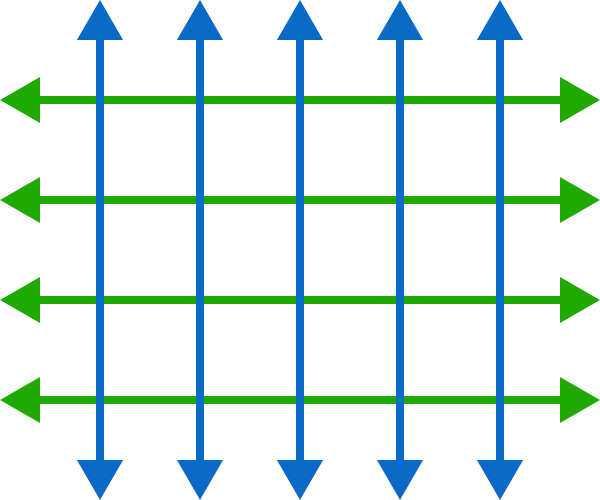# 100 squares

Probability Level 2

In this diagram, there are twelve $1\times 1,$ six $2\times 2,$ and two $3\times 3$ squares for a total of 20 squares.What is the least number of lines needed in total to have exactly 100 squares?

×